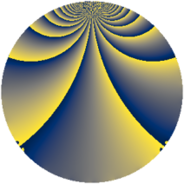# Properties

 Label 1045.2.zLevel $1045$ Weight $2$ Character orbit 1045.z Rep. character $\chi_{1045}(94,\cdot)$ Character field $\Q(\zeta_{10})$ Dimension $464$ Sturm bound $240$

# Related objects

## Defining parameters

 Level: $$N$$ $$=$$ $$1045 = 5 \cdot 11 \cdot 19$$ Weight: $$k$$ $$=$$ $$2$$ Character orbit: $$[\chi]$$ $$=$$ 1045.z (of order $$10$$ and degree $$4$$) Character conductor: $$\operatorname{cond}(\chi)$$ $$=$$ $$1045$$ Character field: $$\Q(\zeta_{10})$$ Sturm bound: $$240$$

## Dimensions

The following table gives the dimensions of various subspaces of $$M_{2}(1045, [\chi])$$.

Total New Old
Modular forms 496 496 0
Cusp forms 464 464 0
Eisenstein series 32 32 0

## Trace form

 $$464 q + 100 q^{4} - 8 q^{5} - 20 q^{6} - 120 q^{9} + O(q^{10})$$ $$464 q + 100 q^{4} - 8 q^{5} - 20 q^{6} - 120 q^{9} - 18 q^{11} - 132 q^{16} - 10 q^{19} - 60 q^{24} + 8 q^{25} - 96 q^{26} - 40 q^{30} - 30 q^{35} + 40 q^{36} - 20 q^{39} + 16 q^{44} - 2 q^{45} - 98 q^{49} + 79 q^{55} - 30 q^{61} + 108 q^{64} - 108 q^{66} - 20 q^{74} + 12 q^{80} + 56 q^{81} + 125 q^{85} - 35 q^{95} - 40 q^{96} + 158 q^{99} + O(q^{100})$$

## Decomposition of $$S_{2}^{\mathrm{new}}(1045, [\chi])$$ into newform subspaces

The newforms in this space have not yet been added to the LMFDB.